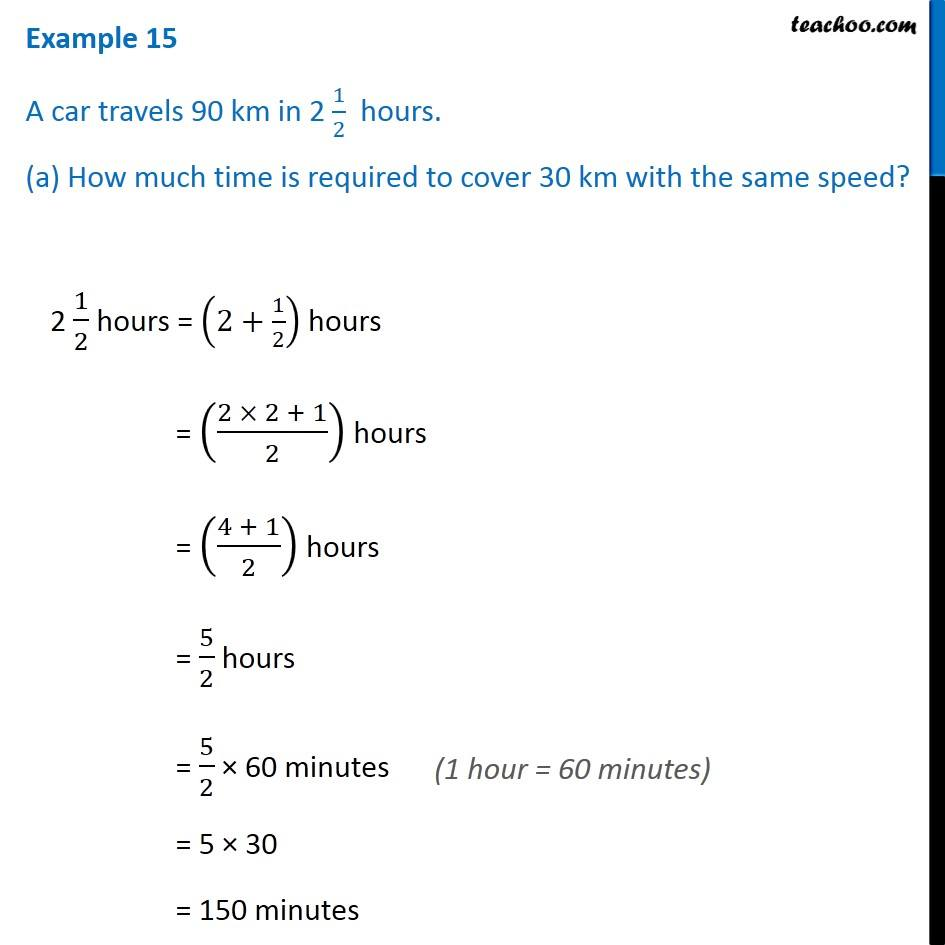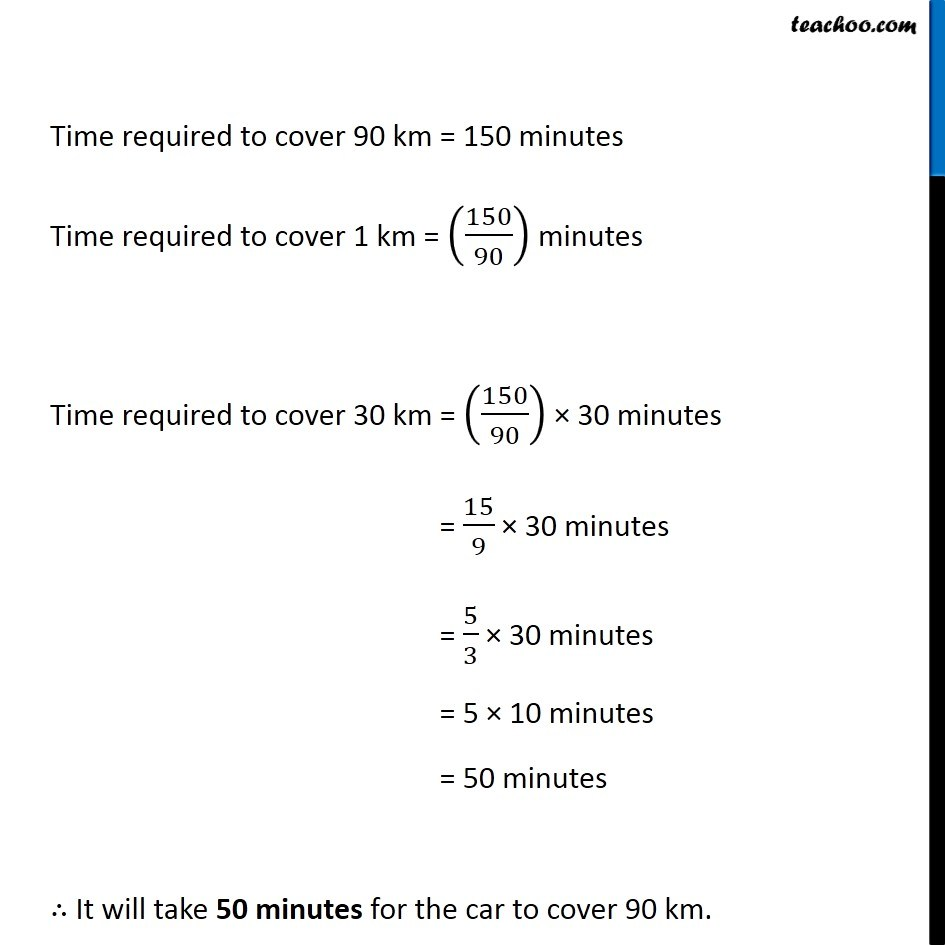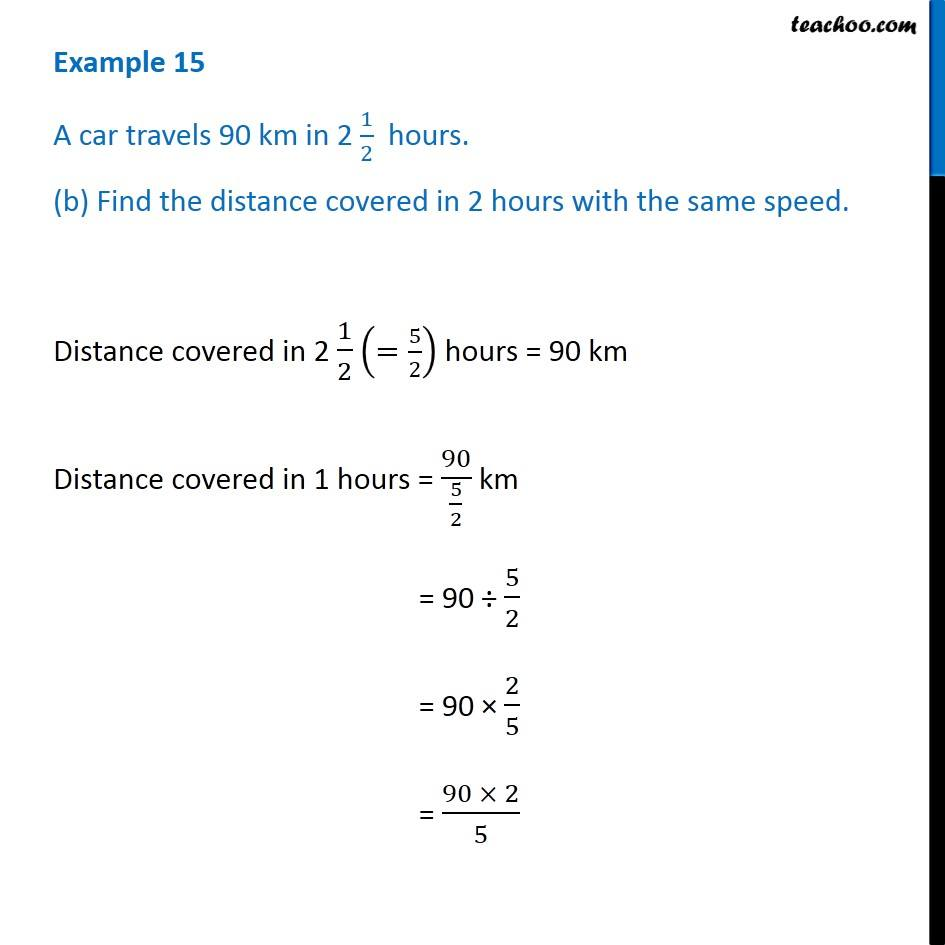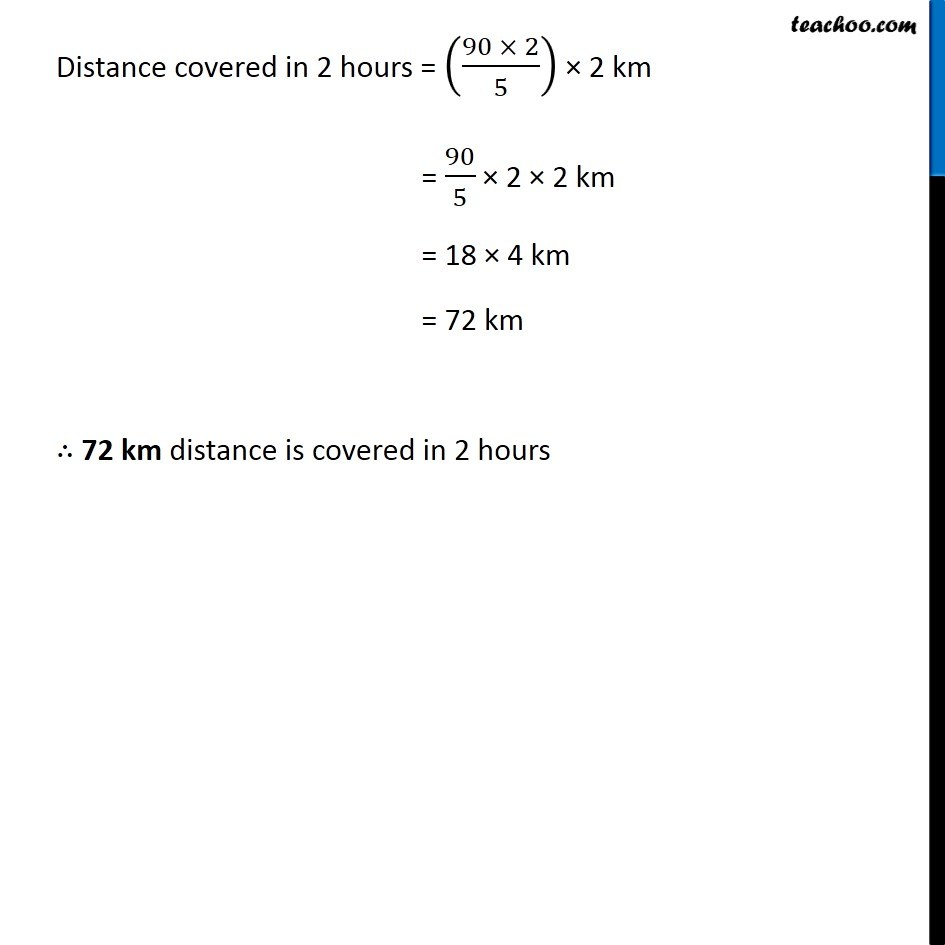Unitary Method

Chapter 12 Class 6 Ratio And Proportion
Concept wise### Transcript

Example 15 A car travels 90 km in 2 1/2 hours. (a) How much time is required to cover 30 km with the same speed? 2 1/2 hours = (2+1/2) hours = ((2 × 2 + 1)/2) hours = ((4 + 1)/2) hours = 5/2 hours = 5/2 × 60 minutes = 5 × 30 = 150 minutes Time required to cover 90 km = 150 minutes Time required to cover 1 km = (150/90) minutes Time required to cover 30 km = (150/90) × 30 minutes = 15/9 × 30 minutes = 5/3 × 30 minutes = 5 × 10 minutes = 50 minutes ∴ It will take 50 minutes for the car to cover 90 km. Example 15 A car travels 90 km in 2 1/2 hours. (b) Find the distance covered in 2 hours with the same speed. Distance covered in 2 1/2 (=5/2) hours = 90 km Distance covered in 1 hours = 90/(5/ 2) km = 90 ÷ 5/2 = 90 × 2/5 = (90 × 2)/5 Distance covered in 2 hours = ((90 × 2)/5) × 2 km = 90/5 × 2 × 2 km = 18 × 4 km = 72 km ∴ 72 km distance is covered in 2 hours